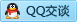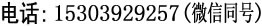首页 公司简介 产品介绍 电容知识 售后服务 联系我们 Engliash

# CBB61型金属化聚丙烯薄膜介质电容器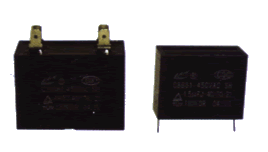1.矩形阻燃塑料外壳，体积小，重量轻，损耗角正切小，有良好的自愈性。

2.适用于电冰箱、电风扇、电动缝纫机、空调等各种家用电器设备中。

⑴.试验条件

⑵.实验后性能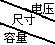250VA.C. 350VA.C. 400VA.C. 500VA.C. L×B×H L×B×H L×B×H L×B×H 1 32×13×22 32×15×30 32×15×30 37×14×26 1.2 32×13×22 32×15×30 *47×14×28 37×18×28 1.5 32×15×30 47×14×28 *47×14×28 37×18×28 2 32×15×30 47×14×28 47×18×34 47×18×34 2.5 47×14×28 47×18×34 47×18×34 47×18×34 2.75 47×14×28 47×18×34 47×22×34 47×18×34 3 47×14×28 47×18×34 47×22×34 47×22×34 3.5 47×14×28 47×18×34 47×22×34 47×22×34 4 47×18×34 47×22×34 47×28×34 47×28×34 4.5 47×18×34 47×22×34 47×28×45 47×28×34 4.75 47×18×34 47×28×34 47×28×45 5 47×18×34 47×28×34 47×28×45 6 47×22×34 47×28×45 47×28×45 8 47×28×34 47×28×45 48×33×49 10 47×28×45 48×33×49 70×38×44 12 47×28×45 16 50×27×40 20 47×33×49 32 70×38×44

*也可为37×14×26外壳（B式）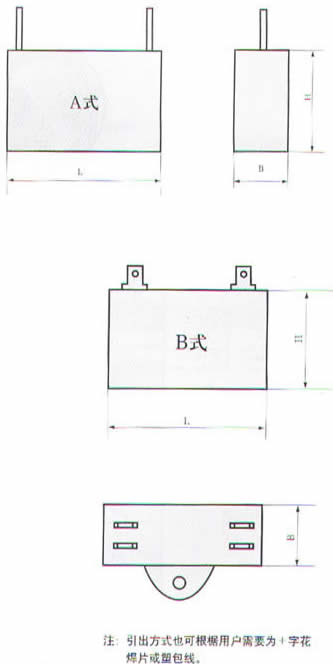类别 直插 结构 固定 封装外形 长方形 特性 旁路 引出线类型 同向引出线 标称容量 1μF-32μF

## 聚丙烯薄膜电容器相关链接：